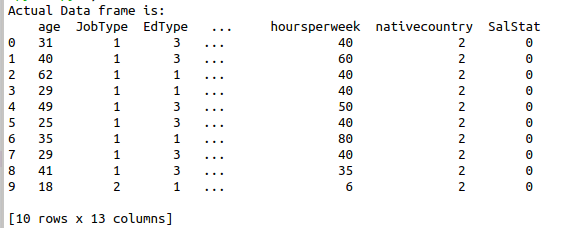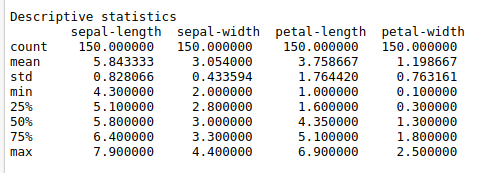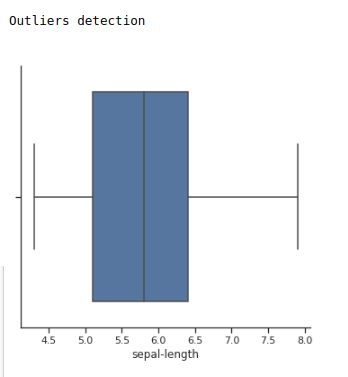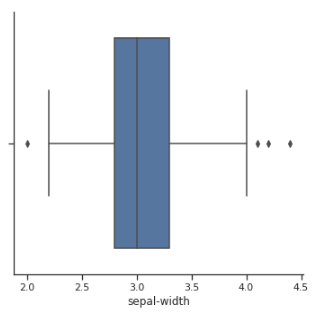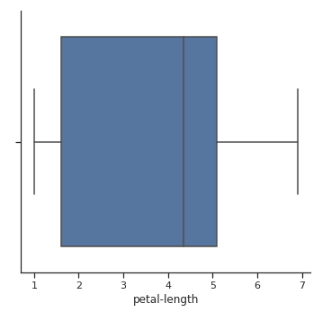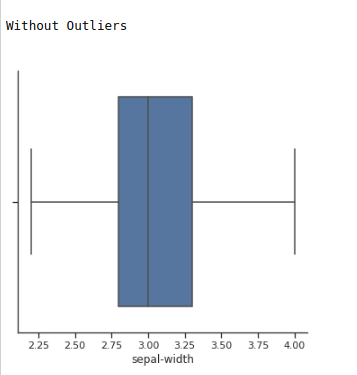• #5, First Floor, 4th Street Dr. Subbarayan Nagar Kodambakkam, Chennai-600 024 Landmark : Samiyar Madam
• pro@slogix.in
• +91- 81240 01111

Income prediction for the given data set using python?

Description

To predict individuals income using Logistic Regression in python.

Process

Get the data set(population).

Clean the data.(population).

Find and fill the missing values(population).

Check outliers(for dependent variables).

After fill missing values take random sample from the population.

Write the sample data into a CSV file (Easy to handle).

Read the sample data from CSV file.

Make it as a data frame.

Check if there is any missing values.

Calculate basic descriptive statistic for sample data.

Check correlation of whole data frame.

Take the variables which has the highly correlated with y(target) variable.

Correlation range must lies in between -1 to 1.

Take X variables and y variable.

Split X and y into train and test data sat.

Import logistic regression from sklearn library.

Build the regression model.

Fit the X_train and y_train data in to the model.

Make predictions.

Calculate the coefficients, intercept, confusion matrix by using sklearn.

metrics library.

Based on the confusion matrix we can calculate the accuracy,specificity and sensitivity also.

Sapmle Code

#import libraries

import pandas as pd

from sklearn.model_selection import train_test_split

from sklearn.linear_model import LogisticRegression

from sklearn import metrics

from sklearn.metrics import classification_report

import numpy as np

import matplotlib.pyplot as plt

import seaborn as sns

#Take sample from population

Sathish/Spyder workings/sample.csv’)

df=pd.DataFrame(data)

#checking missing values

print(“Checking missing values in the sample”)

print(df.isnull().sum())

print(“\n”)

print(“Descriptive statistics”)

print(df.describe())

print(“\n”)

print(“Correlation is”)

print(df.corr(method=’pearson’))

#Depends upon the correlation choose X variable

X=df[[‘hoursperweek’,’relationship’,’EdType’]]

#Fix the target variable

y=(df[‘SalStat’])

#Split the data into train and test data

X_train, X_test, y_train, y_test = train_test_split(X, y, test_size=0.25, random_state=0)

print(“Shape of train data of X\n”,X_train.shape)

print(“Shape of train data of y\n”,y_train.shape)

#build the model

logmodel = LogisticRegression()

result=logmodel.fit(X_train,y_train)

#predictions

y_pred = logmodel.predict(X_test)

df1=pd.DataFrame({‘Actual’:y_test, ‘Predicted’: y_pred})

print(df1)

#regression c-efficients and intercept

print(“Regression intercept is”,logmodel.intercept_)

print(“Regression coefficient is”,logmodel.coef_)

#classification report

print(classification_report(y_test,y_pred))

cm = metrics.confusion_matrix(y_test, y_pred)

print(“The confusion matrix is:\n”,cm)

#finding score of the model

print(“Model score”)

score=result.score(X_train,y_train)

print(score)

print(“Accuracy:”,metrics.accuracy_score

(y_test, y_pred))

print(“Precision:”,metrics.precision_score

(y_test, y_pred))

print(“Recall:”,metrics.recall_score(y_test, y_pred))
class_names=[0,1]

fig, ax = plt.subplots()

tick_marks = np.arange(len(class_names))

plt.xticks(tick_marks,class_names)

plt.yticks(tick_marks,class_names)

# create heatmap

sns.heatmap(pd.DataFrame(cm), annot=True, cmap=”YlGnBu” ,fmt=’g’)

ax.xaxis.set_label_position(“top”)

plt.tight_layout()

plt.title(‘Confusion matrix’)

plt.ylabel(‘Actual label’)

plt.xlabel(‘Predicted label’)

Screenshots